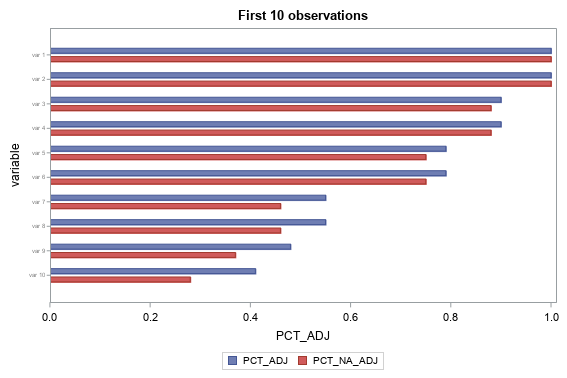## bar plot for last list of variables

Hi,

this may seem like a really simple question but I just cannot get it to work! I have a very large list of variables (~100) with some respective variables (say x and y). I want to simply do a hbar or vbar plot of these but because of the large number of variables either the all the labels are not printed or I get an error message "the left vertical axis could be fit. There was not enough room in the graphs display area to fit the group axis."

I have tried making the font as small as possible, the bar width as small as possible etc but nothing seems to work. Any help would be greatly appreciated

Code:

proc sgplot data=t022;
hbarparm category=variable response=PCT_ADJ / discreteoffset=-0.17 barwidth=0.2;
hbarparm category=variable response=PCT_NA_ADJ / discreteoffset=0.17 barwidth=0.2;
yaxis valueattrs=(color=gray size=0.5pt) offsetmin=0.1 offsetmax=0.1;
run;

4 REPLIES 4

## Re: bar plot for last list of variables

You have 100 variables but your code only refers to 3?

Do you mean observations? Can you show a representative example of your data and expected output.

## Re: bar plot for last list of variables

sorry yes I meant observations.

example of what I am trying to plot:

 Row Labels x var 1 1.00 1.00 var 2 1.00 1.00 var 3 0.90 0.88 var 4 0.90 0.88 var 5 0.79 0.75 var 6 0.79 0.75 var 7 0.55 0.46 var 8 0.55 0.46 var 9 0.48 0.37 var 10 0.41 0.28 var 11 0.39 0.26 var 12 0.38 0.25 var 13 0.38 0.25 var 14 0.38 0.25 var 15 0.38 0.25 var 16 0.37 0.24

## Re: bar plot for last list of variables

And each bar should be one observation? Usually you group them in some manner but you haven't identified a common grouping variable. Or do you expect them to be summarized in some fashion, if so what statistic should be presented. Making a bar chart of 100 observations seems a bit weird to me, you could do a scatter plot or something else that may show a bit better but using a horizontal bar chart would also be possible but you'd need to set the length of the chart to be a long enough to handle the number of bars. You have two values so how would those be representing on a bar chart or are you preparing multiple charts?

## Re: bar plot for last list of variables

You could number the rows (observations) in your dataset, and then when you specify the data= in sgplot, you could specify which rows to plot. In the example below, I add a variable called 'obsnum' to do this.

data t022;
datalines;
var 1    1.00 1.00
var 2    1.00 1.00
var 3    0.90 0.88
var 4    0.90 0.88
var 5    0.79 0.75
var 6    0.79 0.75
var 7    0.55 0.46
var 8    0.55 0.46
var 9    0.48 0.37
var 10    0.41 0.28
var 11    0.39 0.26
var 12    0.38 0.25
var 13    0.38 0.25
var 14    0.38 0.25
var 15    0.38 0.25
var 16    0.37 0.24
;
run;

data t022; set t022;
obsnum=_n_;
run;

title "First 1 observations";

proc sgplot data=t022 (where=(obsnum between 1 and 10));
hbarparm category=variable response=PCT_ADJ / discreteoffset=-0.17 barwidth=0.2;
hbarparm category=variable response=PCT_NA_ADJ / discreteoffset=0.17 barwidth=0.2;
yaxis valueattrs=(color=gray size=0.5pt) offsetmin=0.1 offsetmax=0.1;
run;Discussion stats
• 4 replies
• 364 views
• 0 likes
• 3 in conversation